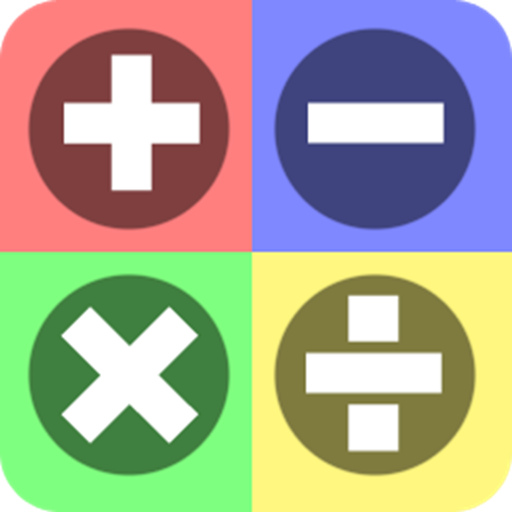# math formulas pack

Version 4.0
2
K
Active installs
Education
Category
5
MB
Size

Math Formulas Pack for students and engineers

with this app you have all math formulas in your mobile

this app contains these formulas:

Algebra: factoring formulas, product formulas, roots formula, powers formula, logarithmic formula, useful equations, complex number, binomial theorem

Geometry: cone, cylinder, isosceles triangle, square, sphere, rectangle, rhombus, parallelogram, trapezoid

Analetical Geometry: 2D coordinate system, circle,hyperbola, ellipse,parabola

Derivation: limits formula, properties of derivative, general derivative formula,trigonometric functions, inverse trigonometric functions,hyperbolic functions, inverse hyperbolic functions

Integration: properties of integration, integration of rational functions, trigonometric functions, hyperbolic functions, exponential and log functions

Trigonometry: basics of trigonometry, general trigonometry formula, sine and cosine rule, table of angle, angle transformation, half double and multiple angle formula, sum of functions, peoduct of functions, powers of functions, Euler's formula, Allied angle table, negative angle identities

Laplace transform: properties of laplace transform, functions of laplace transform

Fourier: fourier series, fourier transform operations, table of fourier transform

Series: arithmetic series, geometric series, finite series, binomial series, power series expansions

Numerical methods: Lagrange, newton's interpolation, newton's forward/backward difference, numerical integration, roots of equation

Vector calculas: vector identities

Probability: basics of probability, expectation, variance, distributions, permutations, combinations

Betta gamma: beta function, gamma function, betta-gamma relation

Z-transform: properties of z transform, some common pairs